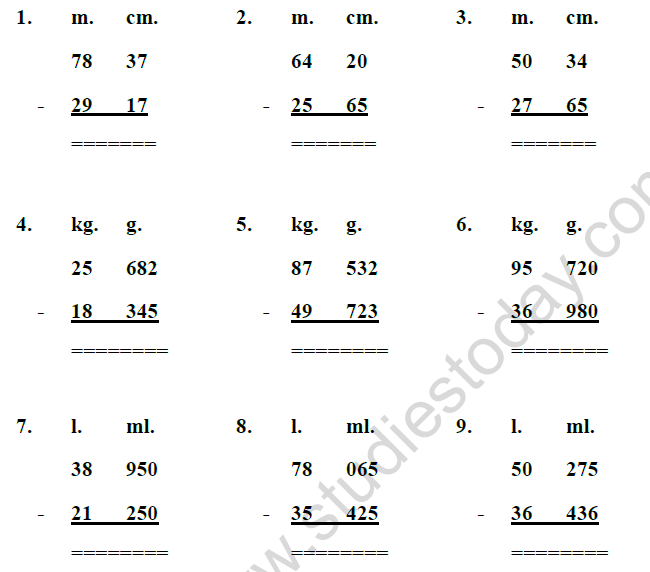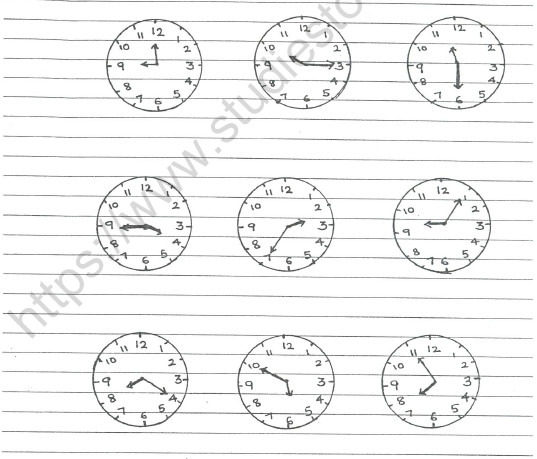# CBSE Class 3 Mathematics Who is Heavier Measurements MCQs

## MCQ for Class 3 Mathematics Who is Heavier Measurements

Class 3 Mathematics students should refer to the following multiple-choice questions with answers for Who is Heavier Measurements in standard 3. These MCQ questions with answers for Grade 3 Mathematics will come in exams and help you to score good marks

### Who is Heavier Measurements MCQ Questions Class 3 Mathematics with Answers

Q 1. Fill in the blanks :

1. The standard unit of measure of length is _________________.

2. 1 kilometer = _________________ meter.

3. 1 Meter = _________________ centimeter.

4. The standard unit for measuring weight is _________________.

5. 1 kilogram = _________________ gram.

6. The weight of an object is expressed in _________________ or grams.

7. The smaller unit of weight is _________________.

8. The bigger unit of weight is _________________.

9. The kilometre, metre and centimetre are the units of _________________.

10. The standard unit for measuring capacity is _________________.

11. Capacity is measured in terms of ______________ and _______________.

12. 1 liter = _________________ milliliter.

13. 1 day = _________________ hours.

14. 1 hour = _________________ minutes.

15. 1 minute = _________________ seconds.

16. 1 week = _________________ days.

17. 1 year = _________________ months.

18. 1 year = _________________ days.

19. 1 leap year = _________________days.

20. The short hand in a clock is the _________________ hand.

21. The long hand in a clock is the _________________ hand.

22. The hour hand completes _________________ rounds in a day.

23. The hour hand takes _________________ hours to complete one round.

24. The minute hand takes _________________ hours to complete one round.

25. The minute hand completes _________________ round in a day.

Q 2. DO AS DIRECTED :Q 3. SubtractQ 4. Convert into Centimetres

1. 5 m

2. 8 m

3. 10 m

4. 25 m

5. 1 m

6. 15 m

Q 5. Convert into metres

1. 9 km

2. 16 km

3. 35 km

4. 8 km

5. 10 km

6. 13 km

Q 6.  Convert into grams

1. 6 kg
2. 8 kg
3. 12 kg
4. 15 kg
5. 23 kg
6. 45 kg

Q 7.  Convert into milliliters
1. 5 l
2. 15 l
3. 20 l

Q 8. Write the time in two ways.Q 9. Write the time as a.m. or p.m.

1. 8:20 in the morning.

2. 9:00 at night.

3. 12:30 after noon.

4. 5:45 in the evening.

5. 4:05 after mid night.

6. 10:40 before noon.

7. 1 o’clock after mid night.

8. 7:15 in the evening.

9. 11:00 at night.

10 1:35 after noon.